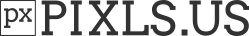# Code Snip to share for rare applications

As I code, I realize there are some cases where there are codes that can be used for obscure application. This will be the thread to share useful code snips that might not have much application.

Right now, I figured a way to implement `pixel_countsort` which works differently, and output differently than the current pixel_sort method. This can be used to have output similar to how python sort things, and it works better for cases of multiple repeating numbers.

``````rep_test:
16,1,1,1,x
+f. int(u(0,5))
+histogram. 100%
f. >begin(n=0;);v=n;n+=i;v;
f.. >v=i(#-1,i);++i(#-1,i);v;
{w#-2},1,1,1
eval... i(#-1,i)=i(#-4,x);
rm[-4--2]
``````

How this work is that it count histogram, then find the minimum index, and then return the index of where it should be. Index are incremented to get the correct places.